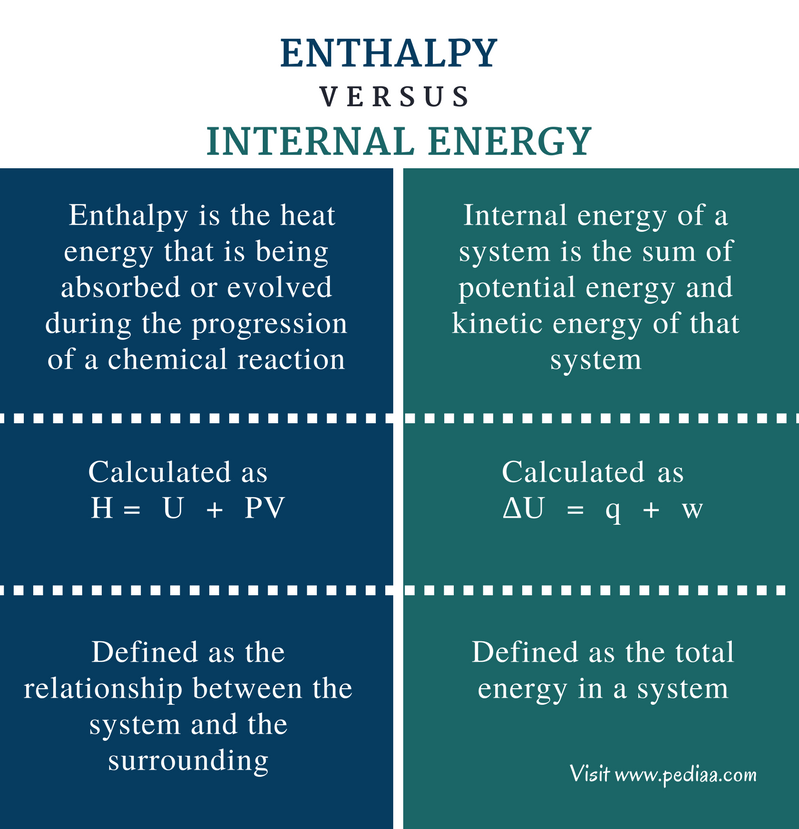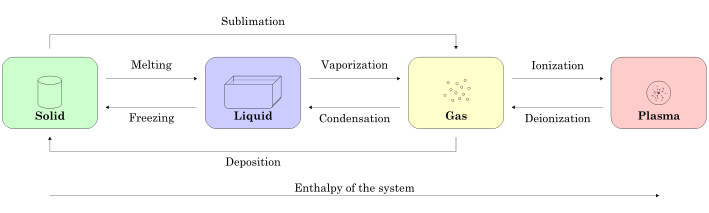# Difference Between Enthalpy and Internal Energy

## Main Difference – Enthalpy vs Internal Energy

Energy can be exchanged between systems and their surroundings in different. Enthalpy and internal energy are thermodynamic terms that are used to explain this energy exchange. Enthalpy is the sum of internal energy types. The internal energy can be either potential energy or kinetic energy. The main difference between enthalpy and internal energy is that enthalpy is the heat absorbed or evolved during chemical reactions that occur in a system whereas internal energy is the sum of potential and kinetic energy in a system.

### Key Areas Covered

1. What is Enthalpy
– Definition, Units, Formula for Calculation, Properties, Examples
2. What is Internal Energy
– Definition, Formula for Calculation, Properties, Examples
3. What is the Difference Between Enthalpy and Internal Energy
– Comparison of Key Differences

Key Terms:  Enthalpy, Heat, Internal Energy, Heat of Fusion, heat of vaporisationJoules, Kinetic Energy, Potential Energy, System, Thermodynamic## What is Enthalpy

Enthalpy is the heat energy that is being absorbed or evolved during the progression of a chemical reaction. The enthalpy is given the symbol H. H indicates the amount of energy. The change of enthalpy is given as ∆H where the symbol ∆ indicates the change of enthalpy. The enthalpy is given in joules (j) or kilo joules (kj).

We can say that enthalpy is the sum of the internal energy of a system. This is because the internal energy is changed during a chemical reaction and this change is measured as the enthalpy. The enthalpy of a process that occurs at a constant pressure can be given as below.

### H = U + PV

Where,

H is the enthalpy,
U is the sum of the internal energy
P is the pressure of the system
V is the volume of the system

Therefore, enthalpy is actually the sum of internal energy and the energy required to maintain the volume of a system at a given pressure. The term “PV” indicates the work that has to be done on the environment in order to make space for the system.

The enthalpy change indicates whether a particular reaction is endothermic or exothermic reaction. If the value of ∆H is a positive value, the reaction is endothermic. That means energy should be given to that system from outside for the reaction to occur. But if the ∆H is a negative value, it indicates that the reaction releases energy to the outside.

Furthermore, enthalpy change occurs in the change of phase or state of substances. For example, if a solid is converted to its liquid form, enthalpy is changed. This is called the heat of fusion. When a liquid is converted to the gaseous form, the enthalpy change is called the heat of vaporisation.Figure 01: The change in state or phase of substances

The above image shows the change in state or phase of a substance in a system. Here, the every transition has its own enthalpy, indicating whether that reaction is endothermic or exothermic.

The temperature of the system has a great influence on the enthalpy. According to the equation given above, enthalpy is changed when the internal energy is changed. When temperature is increased, the internal energy will be increased since the kinetic energy of the molecules is increased. Then the enthalpy of that system is also increased.

## What is Internal Energy

Internal energy of a system is the sum of potential energy and kinetic energy of that system. Potential energy is the stored energy and kinetic energy is the energy generated due to the motion of molecules. The internal energy is given by the symbol U and the change in the internal energy is given as ∆U.

The change in internal energy at a constant pressure is equal to the enthalpy change in that system.  The change in the internal energy can occur in two ways. One is due to heat transfer – the system can absorb heat from outside or can release heat to the surrounding. Both ways can cause the internal energy of the system to be changed. The other way is by doing work. Therefore, the change in internal energy can be given as below.

### ∆U = q + w

Where,

∆U is the change in internal energy,
q is the heat transferred,
w is the work done on or by the system

However, an isolated system cannot have a term ∆U because internal energy is constant and, the energy transfer is zero and no work is done. When the value for ∆U is positive, it indicates that the system absorbs heat from the outside and work is done on the system. When the ∆U is a negative value, then the system release heat and work is done by the system.

However, internal energy can exist as potential energy or kinetic energy but not as heat or work. This is because heat and work only exist when the system undergoes changes.

## Difference Between Enthalpy and Internal Energy

### Definition

Enthalpy: Enthalpy is the heat energy that is being absorbed or evolved during the progression of a chemical reaction.

Internal Energy: Internal energy of a system is the sum of potential energy and kinetic energy of that system.

### Equation

Enthalpy: The enthalpy is given as H =  U  +  PV.

Internal Energy: The internal energy is given as  ∆U  =  q  +  w.

### System

Enthalpy: Enthalpy is defined as the relationship between the system and the surrounding.

Internal Energy: Internal energy is defined as the total energy in a system.

### Conclusion

Enthalpy is related to systems that are in contact with the surrounding and the internal energy is the total energy that a particular system is composed of. However, the change in enthalpy and change in internal energy are very important in determining the type and the nature of the chemical reactions that are going on in a system. Therefore, it is important to clearly understand the difference between enthalpy and internal energy.

##### References:

1.”Enthalpy.” Encyclopædia Britannica. Encyclopædia Britannica, inc., n.d. Web. Available here. 17 July 2017.
2.”How do I distinguish internal energy and enthalpy?” Physical chemistry – Chemistry Stack Exchange. N.p., n.d. Web. Available here. 17 July 2017.

##### Image Courtesy:

1. “Physics matter state transition 1 en” By ElfQrin – Own work, GFDL) via Commons Wikimedia